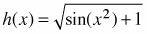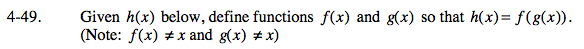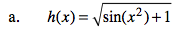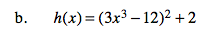### Home > CALC > Chapter 4 > Lesson 4.2.1 > Problem4-49

4-49.
1. Given h(x) below, define functions f(x) and g(x) so that h(x) = f(g(x)). (Note: f(x) ≠ x and g(x) ≠ x) Homework Help ✎

1.2. h(x) = (3x3 − 12)2 + 2One possible solution is:
g(x) = x2

$f(x)=\sqrt{\text{sin}x+1}$

Find another possible solution.One possible solution is:
g(x) = 3x3
f(x) = (x − 12)2 + 2
Find another possible solution.